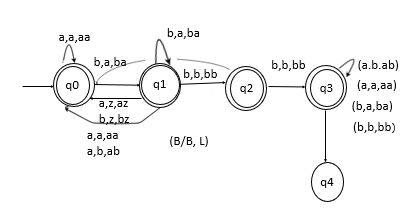# Construct a PDA that accepts (a,b)* language but not contain bbbb?

Push down Automata (PDA) is complement of the PDA that contain Substring bbb

Steps

• Make the PDA for accepting those strings that have conation bbb.

• Complement it by making non-accepting as accepting and vice versa.

Construct PDA

You can construct the PDA as shown below for the (a,b)* languageThe nature of transition format is Input, Top of stack, PUSH/POP

Example

a ,a , aa means on i/p a and top of stack is a then push a

• At q0 i,e initial if a or b anything came move state to q1

• Till q1 we get 1 b to make substring b_ _ so now on q1 if a comes, then it will disturb the pattern of bbb. So make a move to q0, as shown in PDA above.

• But on getting b, b, bb that means i/p is b and Top of stack is b. Push bb and now the pattern is bb_ and move to state q2.

• Now at q2, we get 2 bb to make substring bb_. So now, if at q2 a comes, then it will disturb the pattern of bbb. So make a move to q0, as shown in PDA above.

• But on getting b, b, bb that means i/p is b and Top of stack is b. Push bb and now the pattern is bbb and move to state q4.

• At state q4 now, we have completed the substring bbb. So now, a or b any symbol is accepted.

• When the whole string is ended and state is q4, we move to state q5.

• Now, if we want the language that accepts the string having bbb as a substring, then q5 is the final state.

• But our question is we don't want bbb. So all the other states are final states, that is q0,q1,q2,q4 all are final states.
• So, the PDA is completed.